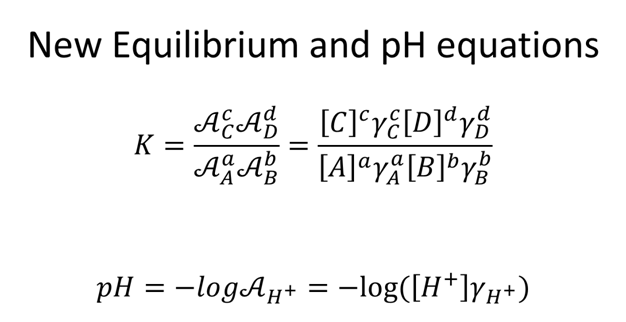# Solubility and Ionic Strength (Fry-Petit)

Homework: Chapter 12: 2, 4, 5, 9-13.b, 14-19• What was the effect of NaI on the solubility of PbI2? Why?

$\ce{PbI_{2(s)} ⇆ Pb^2+ + 2I-}\nonumber$

• What is the effect of adding KNO3 to the above rxn? Why?

STOP• What is the ionic strength of a 0.00333 M solution of PbI2 and the activity coefficient of Pb2+ and I-?

STOP

• Calculate the solubility of 0.00333 M PbI2 (Ksp 7.9×10-9) solution with 2 different scenarios.
• Assume PbI2 solubility is low enough not to effect ionic strength.

• Assume PbI2 solubility effects ionic strength.

• How does the solubility differ and how can you explain the difference?

STOP• Calculate the ionic strength and solubility of a saturated solution of 0.0125 M PbI2 with 0.05 M NaCl. Assume PbI2 solubility effects ionic strength.
• Calculate the ionic strength

STOP

• Interpolate to determine the $$γ_{Pb^{2+}}$$ and $$γ_{I^-}$$

• Determine the solubility